×#### Thank you for registering.

One of our academic counsellors will contact you within 1 working day.

Click to Chat

1800-1023-196

+91-120-4616500

CART 0

• 0

MY CART (5)

Use Coupon: CART20 and get 20% off on all online Study Material

ITEM
DETAILS
MRP
DISCOUNT
FINAL PRICE
Total Price: Rs.

There are no items in this cart.
Continue Shopping```Abnormal Molar Masses

Abnormal Molar Mass

Association of Solute Particles

Dissociation of Solute Particles

Van’t Hoff Factor

Abnormal Molar Mass

Molecular masses of the solute can be easily determined with the help of colligative properties; relative lowering in vapour pressure, boiling point elevation, freezing point depression, and osmotic pressure easily. But, we derived the relation between the molecular mass of solute and colligative properties under some assumptions, which are:

The solution is diluted that is, solvent is available in large amount in order to obey Raoult’s Law

The solute neither went dissociation or association in the solution

So, how do we determine the molecular mass of solute in case the above assumptions are not valid? Basically, the abnormality is due to:

Association of Solute Particles

Dissociation of Solute Particles

Association of Solute Particles

Some solute molecules start to associate inside the solution. This means now there are less number of solute particles in the solution. As colligative properties vary with solute particles in the solution, they will decrease along with the solute particles. As colligative properties are inversely proportional to the molecular mass of solute, we get a higher molar mass of the solute. For Example, ethanoic acid or acetic acid ( CH3COOH) associate in solution to form a dimer due to hydrogen bonding.

Dissociation of Solute Particles

Some solute molecules, generally electrolytes dissociate into two or more ions/particles when dissolved in a solution. This leads to increase in solute particles in the solution, thereby increase in colligative properties of solutions. As colligative properties and molecular mass of solute varies inversely, we get a lower molar mass of the solute.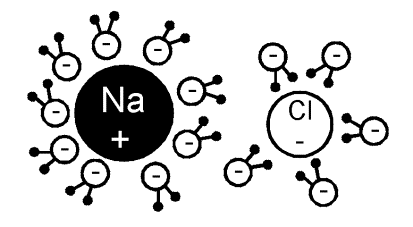Image 1: NaCl dissociate into two ions when dissolved in water

Such a molar mass, whose value is higher or lower than its expected value is known as Abnormal Molar Mass. For Example, weak electrolytes dissociate in water to form ions, example Hydrofluoric Acid (HF), dissociate into hydrogen cation and fluorine anion.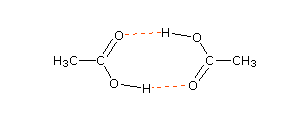Image 2: Ethanoic Acid dimerise due to hydrogen bonding

Van’t Hoff Factor

To determine colligative properties for solutions which undergo association and dissociation, a Dutch physical chemist Jacobus Henricus van’t Hoff in the year 1880 introduced the concept of Van’t Hoff Factor i to sort out association and dissociation problem while calculating the molar mass of solute.

The Van’t Hoff factor is denoted by ‘i’ and is obtained when we divide normal mass with an abnormal mass of solute. Mathematically, it is represented in three forms: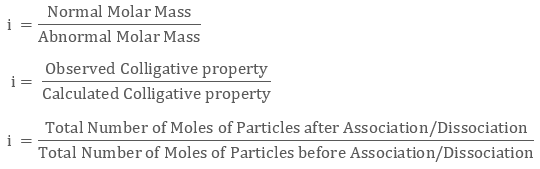Calculation of i:

First, we write an equation of the solute being associated or dissociated

In case of dissociation, i = 1 + (n-1) α where n is number of particles dissociated and α is degree of dissociation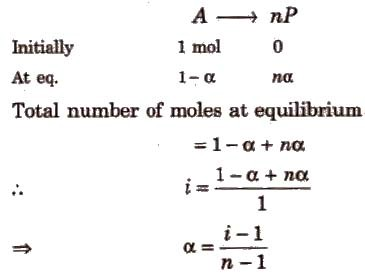Image 3: Derivation of degree of association

In case of association, i = 1 + (1/n – 1) α where n is the number of particles dissociated and α is degree of dissociation, the derivation is as follows: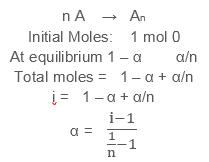For solutes depicting Association, the Van’t Hoff factor is always less than 1, For Example when benzoic acid associates in benzene

For solutes showing dissociation, the Van’t Hoff factor is always greater than 1, For Example, KCl and NaCl both have Van’t Hoff factor 2

For particles showing neither dissociation or association, the Van’t Hoff Factor is taken as 1

The modified equations which can be used in the determination of molar mass in case of association or dissociation are as follows:

Relative Lowering of Vapour pressure of Solvent,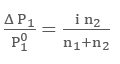where n2 = moles of solute

n1 = moles of solvent

= change in vapour pressure of solvent

P10 = vapour pressure of pure solvent

Boiling Point Elevation, Δ Tb = i Kb m where Kb is elevation constant and m is molality of soluteImage 4: Elevation in boiling point relation

Freezing Point Depression Δ Tf = i Kf m where Kf is depression constant and m is molality of soluteImage 5: Depression in freezing point relation

Osmotic Pressure π = iCRT where C, R and T denotes concentration of solute, universal gas constant and temperature respectively

Example: The freezing point depression of an HF (aq) solution with molality 0.10 m is -0.201 C0. What will be the Van’t Hoff factor? Take the value of Kf to be 1.86 C0m-1

The solution HF (aq) will dissociate as:

HF (aq) → H+ + F-

We have Δ Tf = -0.201 C0 , m = 0.10 and Kf = 1.86 C0m-1

The freezing point depression is Δ Tf = i Kf m

0.201 = i × 1.86 × 0.10

The value of Van’t Hoff factor will be 1.08

Watch this Video for more reference

Abnormal Molar Masses
```### Course Features

• 731 Video Lectures
• Revision Notes
• Previous Year Papers
• Mind Map
• Study Planner
• NCERT Solutions
• Discussion Forum
• Test paper with Video Solution